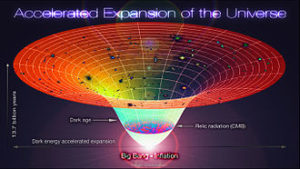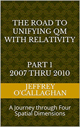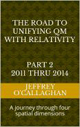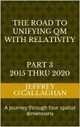# Gravity time dilation and a simple explanation of Dark Energy

Recently observations have suggested a force called Dark Energy is needed to account for the fact the expansion of the universe is accelerating. However. that may be an illusion created by the effect gravity has on time.

Einstein told us and it has been observed the rate at which time is perceived to move is slower in all environments where the gravitational potential is greater with respect where it is being observed. This means the further we look back in time, where universe gravitational potential of the more densely pack matter was greater the estimate of its rate of expansion would be less than it actually was if those effects were not taken into consideration. In other words, if one had not considered the Relativistic slowing of time caused by its gravitational potential that rate would be faster in the past than the present value suggests.However, we also know the gravitational potential has a slowing effect on the universe’s expansion and because that potential decreases as its volume increase, its expansion rate also decreases. In other words, as the universe expands the slowing effect of gravity has on it decreases.

Putting it another way, the rate of universe’s expansion would to appear to occur more rapidly than it actually did from the perspective of present due to the effect gravity has on time while its actual rate would decline due to its gravitational potential as the universe expands.

Therefore, to determine its actual rate of expansion at each point in its history one must not only take into account the gravitational effect on time that would make it appear that it was slower that it actual was but one must also consider how gravity actually causes its expansion rate to decrease.

Yet, because of the non-linear effects between the slowing of time created by universe’s gravitational potential with respect to effects it has on its rate of expansion there will be a point in its history where one will APPEAR to overtake the other.

IN OTHER WORDS, IT IS POSSIBLE THE OBSERVATIONS SUGGESTING THE UNIVERSE EXPANSION IS ACCELERATING MAY BE THE RESULT OF THE FACT THE GRAVITATIONAL POTENTIAL OF THE UNIVERSE CAUSES TIME TO APPEAR MOVE SLOWER IN THE PAST THAN IT ACTUALLY DID.

This gives us a way of validating if the gravitational potential and the slowing effect it has on time is what gives us the IMPRESSION the expansion of the universe is accelerating because it has been observed that about 4 billion years ago the universe’s expansion appears to have change from decelerating to an accelerated phase.

This because one can calculate its actual expansion rate by determining how much time would have appeared to move slower due to the differential gravitational potential between the past and present and use that value to determine its actual rate. If it was found that about 4 billion years ago the effect the slowing of time on our perception of its past expansion rate is less than slowing effect gravity would have on, it would appear as if that rate was accelerating even though it was still decelerating.

Some may say the slowing of time slowing would not affect the timing of the expansion because it is also expanding. However, Einstein define the time dilation only in terms of the affects a differential gravitational potential has on it therefore an expanding universe would not affect it. Some may also say that because the universe is expanding the gravitational potential is expanding and weakening at the same rate therefore when we look back the effects it will have on the timing of its expansion will cancel. However, Einstein tells us the timing of events that cause the universe to expand is locked in the past along with its gravitational potential at the time the expansion took place. Therefore, one must take into account the differential gravitational potential between the past and present universe when defining its expansion.

Some have also suggested Relativistic properties space have already been accounted for in the Friedman model that was used in part by scientist to define the accelerated expansion of the universe. However, that is NOT the case because when someone in the past measures the rate of change in the universe’s expansion rate he would NOT need to use it because his entire spatial slice of the universe would be at the same gravitational potential. However this would NOT be the case for someone looking at that spatial slice from the future. He would have to use it because due to the expansion of the universe a differential gravitational potential would have developed between the past and the present. But as the universe expands the rate of change of its expansion will slow because the gravitational density of the universe has decreased while at the same time the relativistic effects defined by Friedman model will also decreased because of the decrease in gravitation density between the past and present. Yet the relativistic properties of space tell us from the perspective of the present the rate of the universe’s expansion is moving faster than it actually is from the perspective of someone who is present at the time when that expansion was taking place. In other words, Friedman equation defines how the rate of the rate of universe’s expansion changes due to a differential gravitational density between a past and present but it does not define the actual rate of from the perspective of someone who was measuring it from his spatial slice. Therefore, to define the ACTUAL rate at which the expansion of the universe is changing from one point to the next one must determine how the change in gravitational densely effects it from the perspective of an observer at each point in its history and NOT with respect to observer who is looking back at it from the present as the Friedman equation does.

 The Road to Unifying QM with Relativity part 1 2007 thru 2010Ebook \$8.00Paper Back \$15.00 The Road to Unifying QM with Relativity part 2 2011 thru 2014Ebook \$8.00Paper Back \$16.00 The Road to Unifying QM with Relativity part 3 2015 thru 2020Ebook \$8.00Paper Back \$18.00